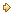Visitors Online: 77 | Sunday 26th May 2019

CBSE Guess > Papers > Question Papers > Class XII > 2004 > Economics > Delhi Set-II

ECONOMICS (Set II—Delhi)

Except for the following questions, all the remaining questions have been asked in Set I.

SECTION - A

Q. 1. Answer the following questions: 1X4
(i) Under which market form, a firm is a price-taker?
(ii) Define marginal cost.
(iii) What causes an upward movement along a supply curve of a commodity?
(iv) Why does the problem of choice arise?

Q. 4. The price elasticity of supply of a commodity is 2.5. At a price of Rs. 5 per unit, its quantity supplied is 300 units. Calculate its quantity supplied at a price of Rs. 4 per unit. 3

Q. 6. What is meant by ‘change in supply’? State three factors that can cause a ‘change in supply’. 4

Q. 8. From the following table, calculate average variable cost of each given level of output: 4

 Output (units) 1 2 3 4 Marginal cost (Rs.) 80 70 72 78

SECTION - B

Q. 13. Answer the following questions: 1X4
(i) The balance of trade shows a deficit of Rs. 5,000 crores and the value of imports are Rs. 9,000 crores. What is the value of exports?
(ii) Is the study of cotton textile industry a macro-economic study or a micro-economic study?
(iii) Define a tax.
(iv) Give two examples of macro studies.

Q. 14. Calculate Gross National Disposable Income from the following data: 3

 Rs. (Crores) (i) Net national product at factor cost (ii) Net factor income from abroad (iii) Consumption of fixed capital (iv) Net indirect taxes (v) Net current transfers from rest of the world 3,000 (-) 50 150 250 300

Q. 16. In an economy, investment expenditure is increased by Rs. 400 crores and marginal propensity to consume is 0.8. Calculate the total increase in income and savings. 3

Q. 17. Distinguish between average propensity to save and marginal propensity to save. The value of which of these two can be negative and when? 3

Q. 22. From the following data calculate Gross National Product Market Price by (i) income method and (ii) expenditure method. 3, 3

 Rs. (Crores) (i) Compensation of employees (ii) Profits (iii) Mixed income of self employed (iv) Rent (v) Interest (vi) Private final consumption expenditure (vii) Net domestic capital formation (viii) Consumption of fixed capital (ix) Net exports (x) Government final consumption expenditure (xi) Net indirect taxes (xii) Net factor income from abroad 400 250 300 80 70 700 120 100 (-) 10 350 60 (-) 10

 Economics 2004 Question Papers Class XII Delhi Outside Delhi Compartment Delhi Compartment Outside DelhiSet ISet ISet ISet ISet IISet IISet IISet IISet IIISet III

CBSE 2004 Question Papers Class XII